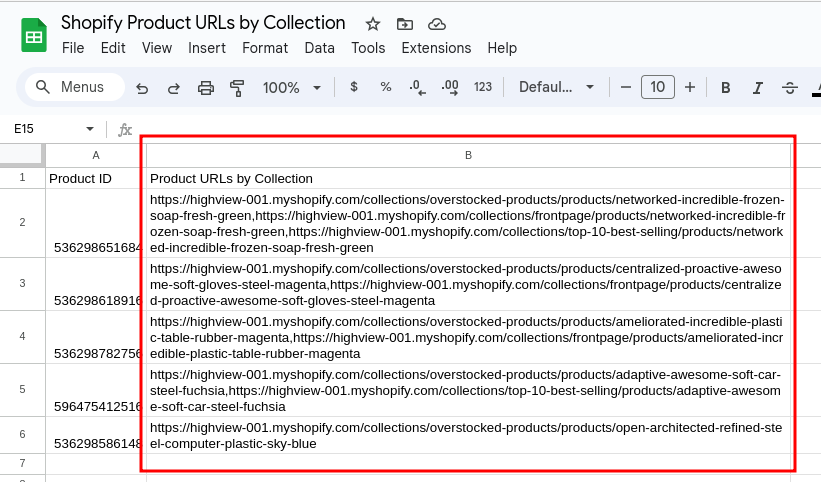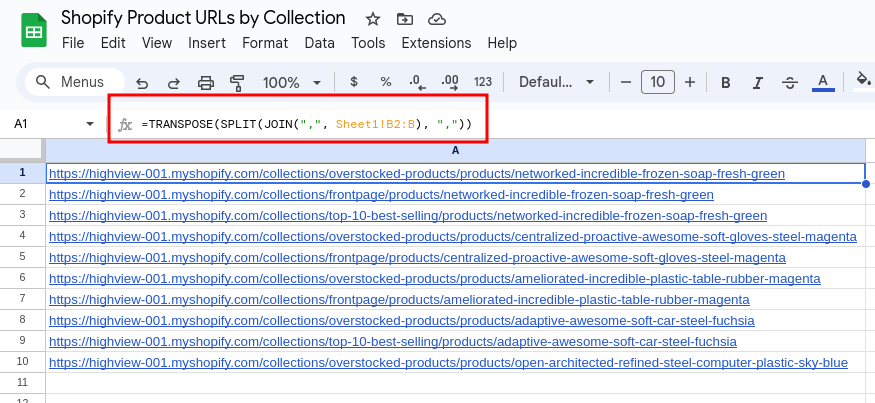## How to Split a Comma-Separated List of URLs From a Column Into Separate Rows in Google SheetsIf you have a spreadsheet that has a column with a comma-separated list of values (like URLs), you can easily combine them and create a separate row for each one in Google Sheets using a formula like this:

`=TRANSPOSE(SPLIT(JOIN(",", Sheet1!B2:B), ","))`

The formula above references column B of Sheet1, where each row's Column B is a comma-separated list of URLs for each product on a Shopify store.

Here's what the formula does:

1. `JOIN(",", Sheet1!B2:B)`: This part of the formula combines all the comma-separated lists of URLs in Column B into a single text string, separating them with a comma (`,`).

2. `SPLIT(..., ",")`: The `SPLIT` function then splits the combined text string into an array of individual URLs, using the comma as the separator.

3. `TRANSPOSE(...)`: Finally, the `TRANSPOSE` function transposes the array of URLs into separate rows.

Here's an example of what the data on Sheet1 looks like, which is basically a list of Shopify product URLs by collection for each product:And here's the output of the formula on Sheet2 (referencing the data from Column B of Sheet1):As you can see, this is a quick and easy way to combine data from one column and then split them up into separate rows for a more readable output.

Related Posts: